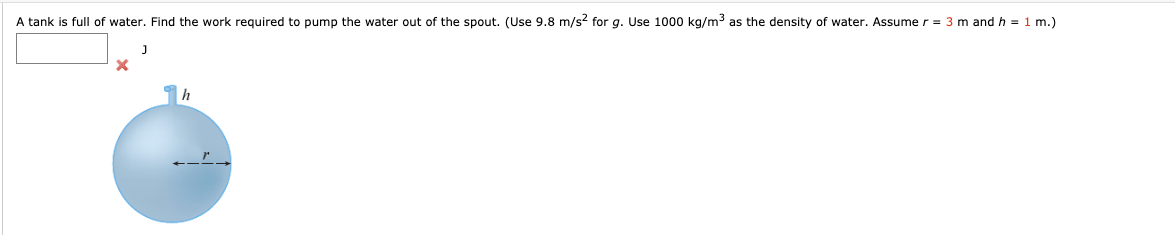# A tank is full of water. Find the work required to pump the water out of the spout. (Use 9.8 m/s? for g.Use 1000 kg/m3 as the density of water. Assume r = 3 mand h = 1m.)

Question
33 viewshelp_outlineImage TranscriptioncloseA tank is full of water. Find the work required to pump the water out of the spout. (Use 9.8 m/s? for g. Use 1000 kg/m3 as the density of water. Assume r = 3 m and h = 1 m.) fullscreen
check_circle

Step 1

It is given that the radius of the spherical tank is 3m and the height of the tank is 1m.

The below figure shows the crossection of the tank.

Step 2

Use the Pythagoras theorem to find the ...

### Want to see the full answer?

See Solution

#### Want to see this answer and more?

Solutions are written by subject experts who are available 24/7. Questions are typically answered within 1 hour.*

See Solution
*Response times may vary by subject and question.
Tagged in

### Calculus